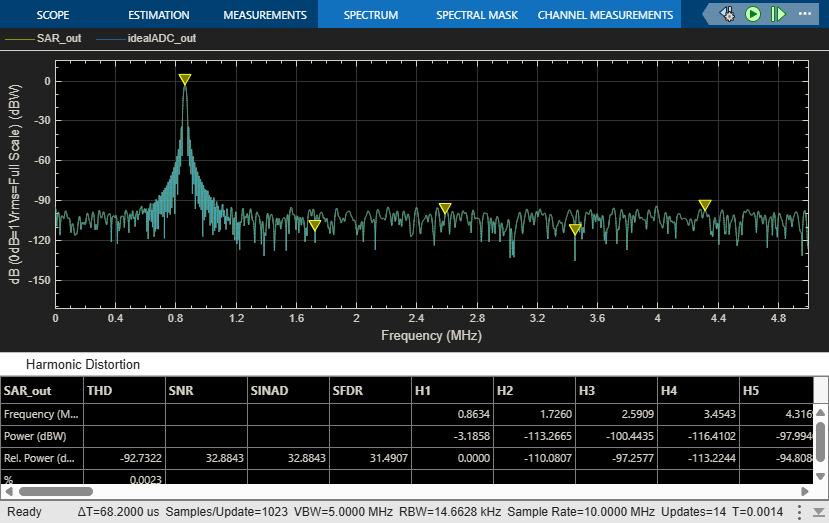This example shows a comparison of the SAR ADC from the Mixed-Signal Blockset™ to the ideal ADC model with impairments presented in Analyzing Simple ADC with Impairments.

This model compares SAR ADC and an equivalent ideal ADC model. To scale the integer output of the SAR ADC to match the floating point output of the ideal ADC, use an output gain and offset. For the comparison to be valid, both ADCs must sample their inputs and then provide their outputs concurrently.

The ideal ADC model is composed of a Quantizer block, a saturation block and a Zero-Order Hold block. The quantization interval is set to be 1 LSB of the SAR ADC. The limits of the saturation block match the SAR ADC's Input range parameter, `[-Vref Vref] / 2`. The Zero-Order Hold and both Sample and Hold blocks sample at Fs, though they do so at differnt times.

The SAR ADC outputs an unsigned, 16-bit integer. The minimum value of the converter's output is 0 and maximum value is 2^ NBits - 1. This is less than the maximum value of the integer as long as the integer has more bits than the converter. The Bias and Scale Results to Volts blocks scale the former integer onto the same range as the converter input and the output of the ideal ADC, by default `-Vref / 2` to `Vref / 2`.

```model = 'SARIdealCompare'; open_system(model); ```The Time Scope block shows the difference between the two outputs on the left and the two outputs themselves on the right.

```open_system([model '/Time Scope']); sim(model); ```The Spectrum Analyzer block shows the spectra of the two outputs.

```close_system([model '/Time Scope']); open_system([model '/Spectrum Analyzer']); sim(model); ```You can substitute the Flash ADC for the SAR ADC in this model to measure its performance.